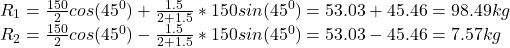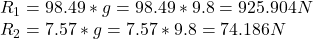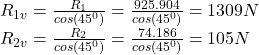## Ross and Chandler are carrying a 150 kg crate up a flight of stairs. The crate is 2.0 m long and 1.5 m high, and its center of gravity is at

Question

Ross and Chandler are carrying a 150 kg crate up a flight of stairs. The crate is 2.0 m long and 1.5 m high, and its center of gravity is at its center. The stairs make a 45.0° angle with respect to the floor. The crate also is carried at an 45.0° angle, so that its bottom side is parallel to the slope of the stairs. If the force each person applies is vertical, what is the magnitude of each of these forces

in progress 0
6 months 2021-07-20T12:25:59+00:00 1 Answers 4 views 0

The magnitude of each force is 1309 N and 105 N

Explanation:

The reaction at each lifter due weight and overturning effect (R₁, R₂) is given as:g = 9.8 m/s²Resolving the reactions to the vertical direction, we get:The magnitude of each force is 1309 N and 105 N